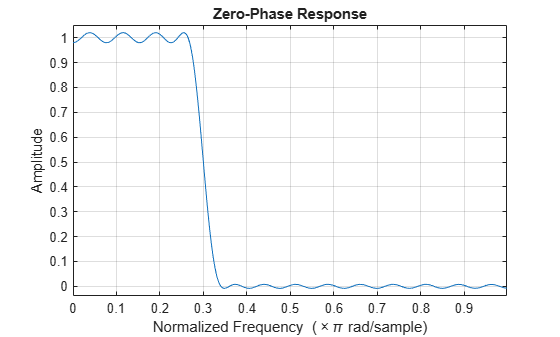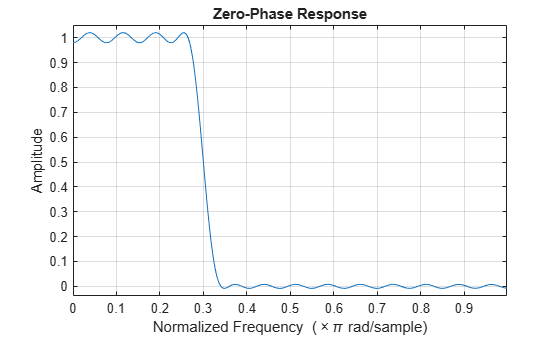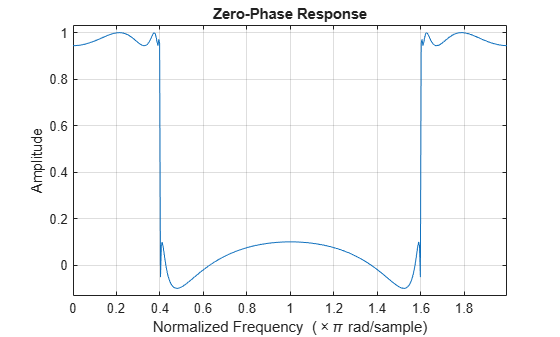Documentation

# zerophase

Zero-phase response of digital filter

## Syntax

```[Hr,w] = zerophase(b,a) [Hr,w] = zerophase(sos) [Hr,w] = zerophase(d) [Hr,w] = zerophase(...,nfft) [Hr,w] = zerophase(...,nfft,'whole') [Hr,w] = zerophase(...,w) [Hr,f] = zerophase(...,f,fs) [Hr,w,phi] = zerophase(...) zerophase(...) ```

## Description

`[Hr,w] = zerophase(b,a)` returns the zero-phase response `Hr`, and the frequency vector `w` (in radians/sample) at which `Hr` is computed, given a filter defined by numerator `b` and denominator `a`. For FIR filters where `a=1`, you can omit the value `a` from the command. The zero-phase response is evaluated at `512` equally spaced points on the upper half of the unit circle.

The zero-phase response, Hr(ω), is related to the frequency response, H(e), by

`$H\left({e}^{j\omega }\right)={H}_{r}\left(\omega \right){e}^{j\phi \left(\omega \right)},$`

where φ(ω) is the continuous phase.

### Note

The zero-phase response is always real, but it is not the equivalent of the magnitude response. The former can be negative while the latter cannot be negative.

`[Hr,w] = zerophase(sos)` returns the zero-phase response for the second order sections matrix, `sos`. `sos` is a K-by-6 matrix, where the number of sections, K, must be greater than or equal to 2. If the number of sections is less than 2, `zerophase` considers the input to be the numerator vector, `b`. Each row of `sos` corresponds to the coefficients of a second order (biquad) filter. The ith row of the `sos` matrix corresponds to ```[bi(1) bi(2) bi(3) ai(1) ai(2) ai(3)]```.

`[Hr,w] = zerophase(d)` returns the zero-phase response for the digital filter, `d`. Use `designfilt` to generate `d` based on frequency-response specifications.

`[Hr,w] = zerophase(...,nfft)` returns the zero-phase response `Hr` and frequency vector `w` (radians/sample), using `nfft` frequency points on the upper half of the unit circle. For best results, set `nfft` to a value greater than the filter order.

`[Hr,w] = zerophase(...,nfft,'whole')` returns the zero-phase response `Hr` and frequency vector `w` (radians/sample), using `nfft` frequency points around the whole unit circle.

`[Hr,w] = zerophase(...,w)` returns the zero-phase response `Hr` and frequency vector `w` (radians/sample) at frequencies in vector `w`. The vector `w` must have at least two elements.

`[Hr,f] = zerophase(...,f,fs)` returns the zero-phase response `Hr` and frequency vector `f` (Hz), using the sampling frequency `fs` (in Hz), to determine the frequency vector `f` (in Hz) at which `Hr` is computed. The vector `f` must have at least two elements.

`[Hr,w,phi] = zerophase(...)` returns the zero-phase response `Hr`, frequency vector `w` (rad/sample), and the continuous phase component, `phi`. (Note that this quantity is not equivalent to the phase response of the filter when the zero-phase response is negative.)

`zerophase(...)` plots the zero-phase response versus frequency. If you input the filter coefficients or second order sections matrix, the current figure window is used. If you input a `digitalFilter`, the step response is displayed in FVTool.

### Note

If the input to `zerophase` is single precision, the zero-phase response is calculated using single-precision arithmetic. The output, `Hr`, is single precision.

## Examples

collapse all

Use `designfilt` to design a 54th-order FIR filter with a normalized cutoff frequency of 0.3π rad/sample, a passband ripple of 0.7 dB, and a stopband attenuation of 42 dB. Use the method of constrained least squares. Display the zero-phase response.

```Nf = 54; Fc = 0.3; Ap = 0.7; As = 42; d = designfilt('lowpassfir','FilterOrder',Nf,'CutoffFrequency',Fc, ... 'PassbandRipple',Ap,'StopbandAttenuation',As,'DesignMethod','cls'); zerophase(d)```Design the same filter using `fircls1`, which uses linear units to measure the ripple and attenuation. Display the zero-phase response.

```pAp = 10^(Ap/40); Apl = (pAp-1)/(pAp+1); pAs = 10^(As/20); Asl = 1/pAs; b = fircls1(Nf,Fc,Apl,Asl); zerophase(b)```Design a 10th-order elliptic lowpass IIR filter with a normalized passband frequency of 0.4π rad/sample, a passband ripple of 0.5 dB, and a stopband attenuation of 20 dB. Display the zero-phase response of the filter on 512 frequency points around the whole unit circle.

```d = designfilt('lowpassiir','FilterOrder',10,'PassbandFrequency',0.4, ... 'PassbandRipple',0.5,'StopbandAttenuation',20,'DesignMethod','ellip'); zerophase(d,512,'whole')```Create the same filter using `ellip`. Plot its zero-phase response.

```[b,a] = ellip(10,0.5,20,0.4); zerophase(b,a,512,'whole')```